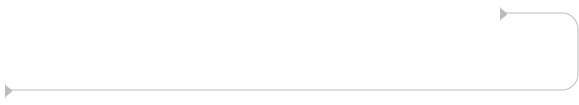Communicate better than your colleagues. All open-source. Get exclusive access.

## What are the different loops in JavaScript?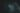Loops are an elementary concept in programming languages. A programmer who understands loops can perform various tasks with ease.

This article will teach us how to repeat a series of actions using loops in JavaScript.

## Prerequisite

• Basic understanding of HTML and JavaScript.

## JavaScript Loops

Loops are an excellent method to make a series of activities repeat themselves. They allow us to repeatedly run a code block until we meet a specific condition. We call this condition the stop condition.

Imagine a situation where we want to print from `1` - `12`. So we `console.log` each number twelve times. That's a lot of work, right?. Using loops in a situation like this will save us time and allow us to write fewer lines of code. That is the primary benefit of using loops.

## Types of Loops

The most common loops in JavaScript are:

• For loop
• For-of loop
• For-in loop
• While loop
• Do-while loop

## For Loops

We use a for loop to repeat a series of actions until a specific condition is false. When a stop condition is true, the for loop runs, and when it is false, it does not.

An example of the for loop syntax is as follows:

``````    for (initial expression; stop condition or counter; increment expression) {
// code block to be executed
}``````

There are three essential expressions in a for loop, separated by semicolons `;`.

• Initial expression: This expression starts the loop. It also allows us to declare a counter or loop variable.
• Stop condition: An initial expression must meet a stop condition so that the loop will run. The code will run if the stop condition is true and terminate if it’s false. If we fail to provide the stop condition, it defaults to true. We call the point at which the condition becomes false the stop condition.
• Increment expression: The increment expression is also called the update expression. We use the increment expression to either increase or decrease the counter. It can also change to a decrement expression when we want a loop to run backward.
• The loop body is the code block we run within a loop.

We use a for loop when we know how many times the loop will run before it starts running.

Example 1: Print from 1 - 12.

``````    for (let i = 1; i <= 12; i++) {
console.log(i);
}

// returns => 1 2 3 4 5 6 7 8 9 10 11 12 ``````

### Let's take a closer look at example 1:

• The initial expression also called the loop variable, is `let i = 1`, which means the loop will start running at `1`. The letter `i` stands for index. It is a common naming convention used for loops. `i` can be anything. It could be `let j = 1`, or `let counter = 1`, for example.
• The stop condition is `i <= 12`. The loop will run as long as the loop variable is less than or equal to `12`. The loop stops when the condition is greater than `12`. For more information on JavaScript comparisons and logical operators, click here.
• `i++` represents the increment expression. The loop variable value will increase from `1` to `2` to `3` until it reaches `12`. After each loop runs, the value of the loop variable will increase by `1`.

Remember to keep the loop variable names consistent. There should be one variable name for increment expression, loop variable, and stop condition.

## How to run a backward For Loop

What if we want a situation where our example 1 runs backward from 12 - 1?. What do we do?.

• We set the loop variable value to the highest specific value in the initial expression.
• We set the stop condition to be less than the loop variable value.
• The increment expression changes to decrement expression. After each loop runs, the value of the loop variable will decrease by `1`.

P.S. The term "incrementing" refers to adding one to a variable's value. Decrementing a variable is the process of subtracting one from its value.

Example 2: Print from 1 - 12 backward.

``````    for (let backwardExp = 12; backwardExp >= 1; backwardExp--) {
console.log(backwardExp);
}

// 12 11 10 9 8 7 6 5 4 3 2 1``````

## How to fix Infinite Loops

An infinite loop is a loop that never ends. The stop condition evaluates to `true` every time, making the loop run indefinitely.

Example 3: Infinite loop

``````    for (let i = 10; i >= 5; i++) {
console.log(i);
}``````

Infinite loops consume a lot of memory on a computer. Make sure the stop condition becomes `false` at some point to avoid writing an endless loop.

When we want to exit from an infinite loop in an exercise, we first refresh the web page and fix the loop.

## Looping through Arrays

Arrays store data types like personal contacts, shopping lists, etc. They are zero-indexed, which means the first item in an array has an index of 0. We can use JavaScript for loops to repeat an action for each item in an array. For more information on JavaScript arrays, click here.

With for loops in arrays, we must use the `.``length` property as the stop condition when looping through each item in the array. The `.length` property allows us to run the loop as many times as the array items.

Example 4:

``````    let myList = ["laptop", "frontend job", "dog"];
for (let i = 0; i < myList.length; i++) {
console.log("I want a " + myList[i]);
}``````

In the above example, the result will be:

``````    "I want a laptop"
"I want a frontend job"
"I want a dog"``````

## How to nest For Loops

In a nutshell, nested loops are when one loop runs inside another. We use nested loops most time to compare elements or items in two Arrays.

Example 5: A nested for loop

``````    // first loop
for (let i = 1; i < 4; i++) {
console.log(`outer loop: \${i}`);

// nested loop
for(let j = 1; j <=2; j++) {
console.log(inner loop: \${j});
}
}``````

In the above example, the result will be:

``````    "outer loop: 1"
"inner loop: 1"
"inner loop: 2"

"outer loop: 2"
"inner loop: 1"
"inner loop: 2"

"outer loop: 3"
"inner loop: 1"
"inner loop: 2"``````

This also applies to nested arrays.

## For Of Loops

The for of loop is one of the newest versions of loops in JavaScript ES6. We use the `for of` loop to run or iterate over arrays or other iterable objects. Iterables are objects we can run over, such as arrays, sets, strings, maps, etc. For more information on iterable objects, click here.

An example of the for of loop syntax is as follows:

``````    for (variable of Iterable) {
// code to be executed
}``````

In the above syntax:

• The variable is the `var`, `let`, or `const` keywords we used to declare the variable.
• The iterable can be an array, string, map, set, etc.

Example 6: Looping through Arrays with the For of Loop.

``````    let codeLine = ['coding', 'design', 'advocate', 'create'];
for (let myCode of codeLine ) {
console.log(myCode);
}``````

In the above example, the result will be:

``````    "coding"
"design"
"create"``````

In Example 6:

• We assign the array's `codeLine` element to the keyword variable `myCode`.
• The `for of` loop runs over every item in the `codeLine` array.

## For In Loops

We use the `for in` loop to run over the property keys of an object. It runs over only the keys of an object with an enumerable value of `true`.

Use the `property.enumerable` function to see if a property is enumerable. It will return `true` or `false`. For more information on enumerable properties, click here.

For in loops can be used on objects, strings and arrays.

An example of the for in loop syntax is as follows:

``````    for (variable in object) {
// code block to be executed
} ``````

In the above syntax:

• The variable is the `var`, `let`, or `const` keywords we used to declare the variable.
• The object refers to the object whose properties we want to run or iterate over.

Example 7: Using the For in Loop with objects.

``````    let stack = {
name: 'cess',
stack: 'frontend',
country: 'Nigeria',
languages: 2,
};

// To get the object keys
for (let profile in stack) {
console.log(profile);
}
// To get the values of the object keys
for (let profile in stack) {
console.log(stack[profile]);
}``````

In the above example, the result will be:

``````    // Output for the object keys
"name"
"stack"
"country"
"languages"

// Output for the values of the object keys
"cess"
"frontend"
"Nigeria"
2``````

In the above example, we used the `for in` loop to run over and print all the `stack` object properties.

• We assign `profile` to the variable key.
• We use my `stack[profile]` to access the value of the object key.

Although using the `for in` loop for arrays is possible, it is generally not recommended. It loops over an object's properties in whichever order it wants, i.e., an arbitrary order. Thus, our arrays may not display in a consistently arranged order.

NOTE: The best way to run over arrays is to use `for` or `for of` loops.

## The difference between a for of loop and a for in loop

The `for of` and `for in` loops differ primarily in the data they iterate or run over.

• We use the `for of` loop to run or iterate over iterable objects such as arrays, sets, strings, maps, etc.
• We use the `for in` loop to run over objects. It runs over only the keys of an object with an enumerable value of true

## While Loops

The while loop continues to run as long as the stop condition is `true`. It will stop running if the condition resolves to `false`.

An example of the while loop syntax is as follows:

``````    while (stop condition) {
// code block to be executed
} ``````

## When to use a While Loop

We use a while loop when we are unsure of the number of times the loop will run before it starts running.

Example 8: Print from 1 - 6.

``````     let i = 1;
while(i <= 6) {
console.log(i);
i++;
}
// returns 1 2 3 4 5 6``````

Here,

• The loop starts running at `1`.
• The while loop evaluates the stop condition inside the parenthesis `(1 <= 6)`. If the stop condition is `true`, it increases by one until it turns `false`.

## The Break statement

The break statement is also known as the loop control statement. We use the break statement to stop a loop from running or, in other words, break out of a loop.

## Do-while Loops

Do-while loops run a block of code (loop's body) at least once before rerunning if the stop condition is `true` or `false`.

An example of the do-while loop syntax is as follows:

``````    do {
// code to be executed
} while(stop condition)``````

### A look at the do-while loop syntax:

• The body of the loop runs first, then the stop condition.
• If the stop condition is `true`, the loop's body runs again until it becomes `false`.

Example 9: Print from 1 - 6

``````    let i = 1;
do {
console.log(i);
i++;
} while(i <= 6)

// returns => 1 2 3 4 5 6``````

## What is the difference between a while loop and a do-while loop?

The while loop checks if the condition‘s resolution before running, whereas the do-while loop runs the code block first before checking for the stop condition.

## Conclusion

In this article, we have learned the concept of loops in JavaScript. The use of loops makes our code more efficient and avoids repeating many lines of code.

## Resources

The following resources may be of help: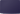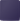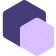Do you have an interest in technical writing?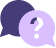## Have any feedback or questions?

We’d love to hear from you.